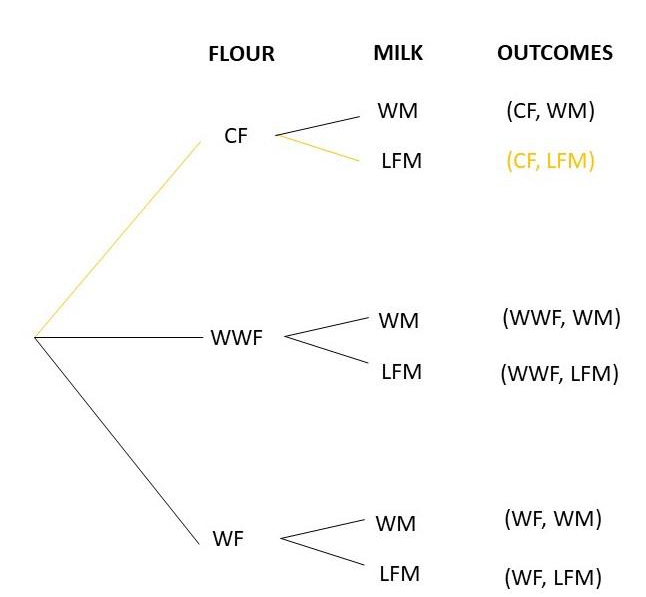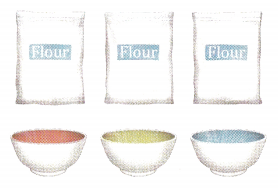# Math in Focus Grade 8 Chapter 11 Lesson 11.2 Answer Key Probability of Compound Events

This handy Math in Focus Grade 8 Workbook Answer Key Chapter 11 Lesson 11.2 Probability of Compound Events detailed solutions for the textbook questions.

## Math in Focus Grade 8 Course 3 B Chapter 11 Lesson 11.2 Answer Key Probability of Compound Events

### Math in Focus Grade 8 Chapter 11 Lesson 11.2 Guided Practice Answer Key

Use a possibility diagram to find each probability.

Question 1.
Two fair four-sided number dice, each numbered 1 to 4 are rolled together. The result recorded is the number facing down. Find the probability that the product of the two numbers is divisible by 2.
Given,
Two fair four-sided number dice, each numbered 1 to 4 are rolled together.
The result recorded is the number facing down.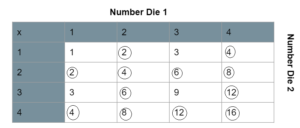3/4

Question 2.
One colored disc is randomly drawn from each of two bags. Both bags each have 5 colored discs: 1 red, 1 green, 1 blue, 1 yellow, and 1 white. Find the probability of drawing a blue or yellow disc.
Given,
One colored disc is randomly drawn from each of two bags. Both bags each have 5 colored discs: 1 red, 1 green, 1 blue, 1 yellow, and 1 white.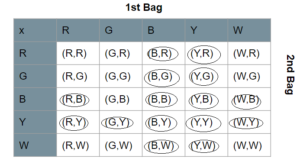R represents red
G represents green
B represents blue
Y represents yellow
W represents white
16/25

Question 3.
A box has 1 black, 1 green, 1 red, and 1 yellow marble. Another box has 1 white, 1 green, and 1 red marble. A marble is taken at random from each box. Find the probability that a red marble is not drawn.
Given,
A box has 1 black, 1 green, 1 red, and 1 yellow marble.
Another box has 1 white, 1 green, and 1 red marble.
Marble is taken at random from each box.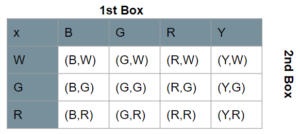B represents black
R represents red
G represents green
Y represents yellow
W represents white
1/2

Question 4.
Three fair coins are tossed together.
a) Draw a tree diagram to represent the possible outcomes.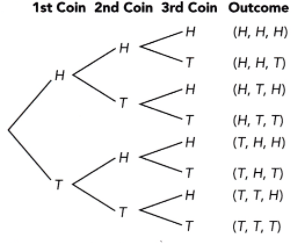T represents tails

Total outcomes = 24
Number of getting all heads = 3
3/24 = 1/8
The probability of getting all heads = 1/8

c) Using your answer in a), find the probability of getting at least two tails.
Total outcomes = 24
1/2
The probability of getting at least two tails = 1/2

### Math in Focus Course 3B Practice 11.2 Answer Key

Question 1.
A bag contains 2 blue balls and 1 red ball. Winnie randomly draws a ball from the bag and replaces it before she draws a second ball. Use a possibility diagram to find the probability that the balls drawn are different colors.
Given,
A bag contains 2 blue balls and 1 red ball = 3
P(red) = 1/3
P(blue) = 2/3
possible outcomes = 4
Thus the probability that the balls drawn are different colors = 4/6

Question 2.
A letter is randomly chosen from the word FOOD, followed by randomly choosing a letter from the word DOG. Draw a tree diagram to find the probability that both letters chosen are the same.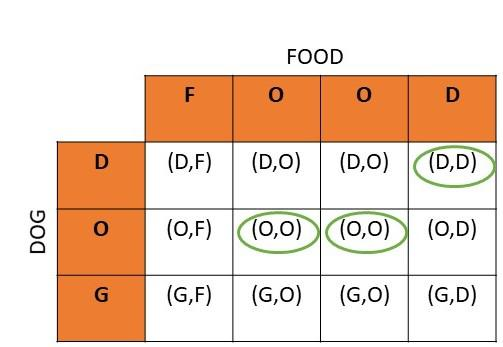There are 12 possible outcomes and 3 among them are favorable outcomes, then
Probability = favorable outcomes/total outcomes
= 3/12
= 1/4
Therefore, the probability of getting two similar letters is 1/4.

Question 3.
Three pebbles are placed in a bag: 1 blue, 1 green, and 1 yellow. First a pebble is randomly drawn from the bag. Then a fair four-sided number die labeled from 1 to 4 is rolled. The result recorded is the number facing down. Use a possibility diagram to find the probability of drawing a yellow pebble and getting a 4.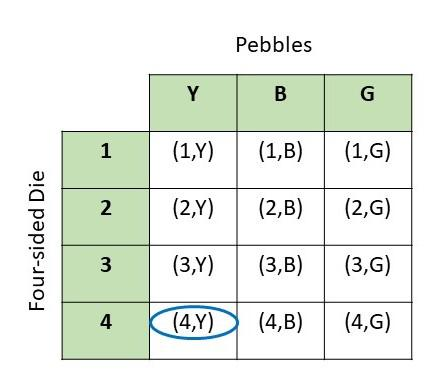As there are 12 possible outcomes and 1 favorable outcome, then
Probability = favorable outcomes/total outcomes
= 1/12
Therefore, the probability of getting (4,y) is 1/12.

Question 4.
Thomas rolled a fair six-sided number die and a fair four-sided number die labeled 1 to 4 together. Use a possibility diagram to find the probability of rolling the number 3 on both.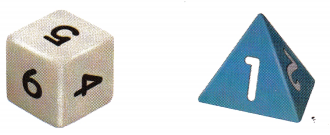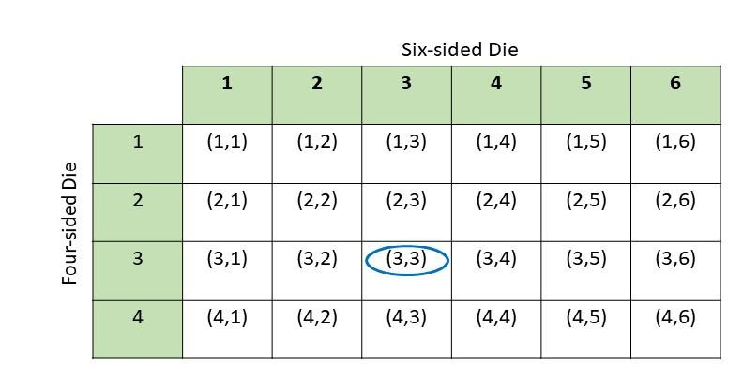As there are total of 24 possible outcomes and 1 favorable outcome, then
Probability = favorable outcome/total outcomes
= 1/24
Therefore, the probability of getting (3,3) is 1/24.

Question 5.
At a bike shop, there are 3 bikes with 20-speed gears and 2 bikes with 18-speed gears. The bike shop also sells 1 blue helmet and 1 yellow helmet. Use a possibility diagram to find the probability of getting an 18-speed bicycle and a blue helmet if randomly selecting one bike and one helmet from among these.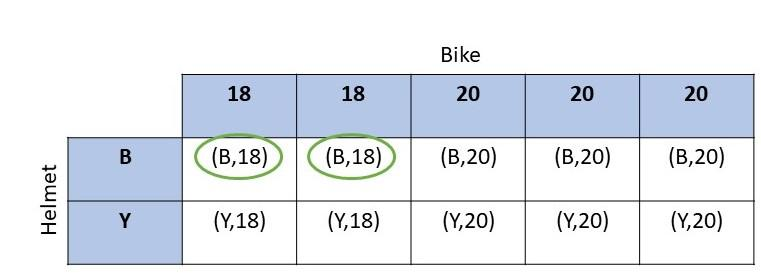As there are 10 possible outcomes and 2 of them are favorable outcomes, then
Probability = favorable outcomes/total outcomes
= 2/10
= 1/5
Therefore, the probability of getting (B,18) is 1/5.

Question 6.
Susan randomly draws a card from three number cards: 1, 3, and 6. After replacing the card, Susan randomly draws another number card. The product of the two numbers drawn is recorded.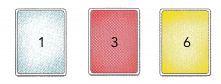a) Use a possibility diagram to represent the possible outcomes.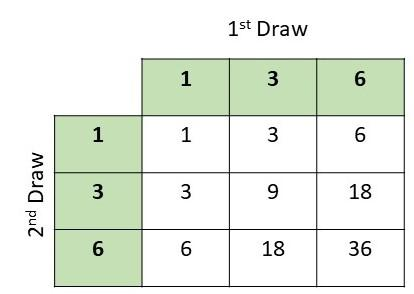b) Using your answer in a), what is the probability of forming a number larger than 10 but less than 30?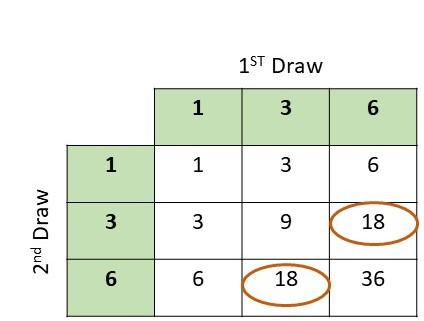There are 9 possible outcomes and 2 of them are favorable outcomes,then
Probability(>10<30) = favorable outcomes/total outcomes
= 2/9
Therefore, the probability of getting greater than 10 and less than 30 is 2/9.

Question 7.
Jane and Jill watch television together for 2 hours. Jane selects the channel for the first hour, and Jill selects the channel for the second hour. Jane’s remote control randomly selects from Channels A, B, and C. Jill’s remote control randomly selects from Channels C, D, and E.
a) Use a possibility diagram to represent the possible outcomes for the channels they watch on television for 2 hours.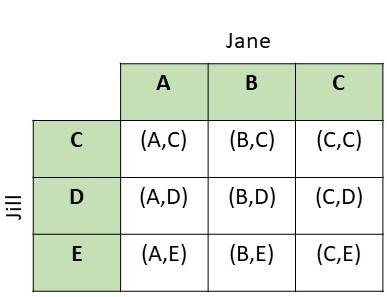b) Using your answer in a), what is the probability of watching the same channel both hours?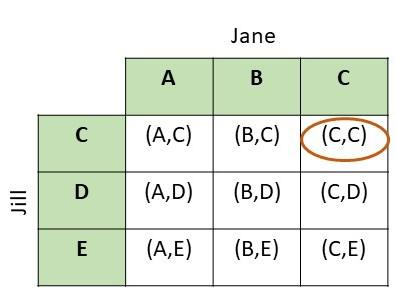As there are 9 possible outcomes and 1 favorable outcome, then
Probability = favorable outcomes/total outcomes
= 1/9

Question 8.
A color disc is randomly drawn from a bag that contains the following discs.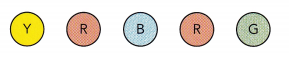After a disc is drawn, a fair coin is tossed. Use a possibility diagram to find the probability of drawing a red disc and landing on heads.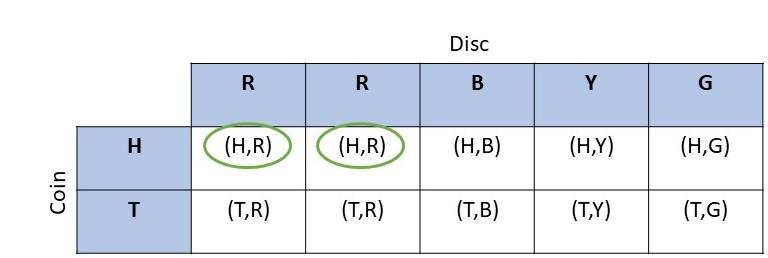As there are 10 possible outcomes and 2 of them are favorable outcomes, then
Probability = favorable outcomes/total outcomes
= 2/10
= 1/5
Therefore, the probability of getting a red disc and head is 1/5.

Question 9.
Karen tosses a fair coin three times. Draw a tree diagram to find the probability of getting the same result in all three tosses.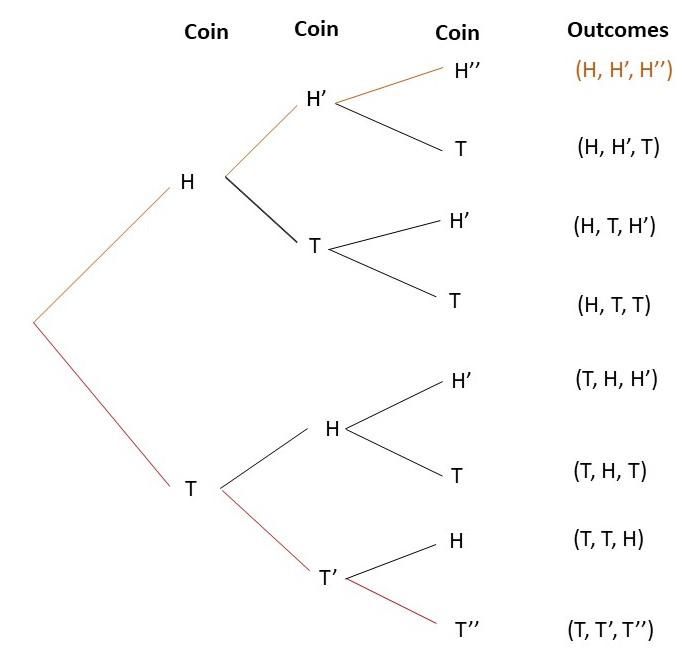As there are total of 8 possible outcomes and 2 favorable outcomes, then
Probability = favorable outcomes/total outcomes
= 2/8
= 1/4
Therefore, the probability of acquiring a (H,H’,H”) and (T,T’,T”) is 1/4.

Question 10.
Mrs. Bridget’s recipes require her to put in some fine maize flour in bowl 1, followed by wheat flour in bowl 2, and rice flour in bowl 3. However, the jars of flour are not labeled, so she randomly guesses which flour to put in which bowl.
a) Draw a tree diagram to represent the possible outcomes.